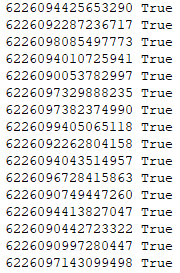# 一、银行卡号码的校验规则

1. 从右到左给卡号字符串编号，最右边第一位是1，最右边第二位是2，最右边第三位是3….

2. 从右向左遍历，对每一位字符t执行第三个步骤，并将每一位的计算结果相加得到一个数s。

3. 对每一位的计算规则：如果这一位是奇数位，则返回t本身，如果是偶数位，则先将t乘以2得到一个数n，如果n是一位数（小于10），直接返回n，否则将n的个位数和十位数相加返回。

4. 如果s能够整除10，则此号码有效，否则号码无效。

#! /usr/bin/python3
# -*- coding: utf-8 -*-

def luhn(card_num):
s = 0
card_num_length = len(card_num)
for _ in range(1, card_num_length + 1):
t = int(card_num[card_num_length - _])
if _ % 2 == 0:
t *= 2
s += t if t < 10 else t % 10 + t // 10
else:
s += t
return s % 10 == 0

if __name__ == '__main__':
print(luhn('6226095711989751'))


# 二、生成符合Luhn规则的银行卡号测试数据

1. 随机生成n-1位字符，称为字符串x。

2. 先假设字符串x有n位（实际上最右边一位缺失是n-1位），将x按照n位长度计算和s，因为最右边第一位是缺失的，忽略跳过，所以计算时最右边一位从2开始。

3. 上一步得到字符串x的校验和s，将s加上一个数字y，使得它正好可以整除10，这个y就是最右边第一位应该放的数字。

4. x+y做字符串拼接运算，得到最终的n位符合Luhn规则的字符串。

#! /usr/bin/python3
# -*- coding: utf-8 -*-

import random

def gen_card_num(start_with, total_num):
result = start_with

# 随机生成前N-1位
while len(result) < total_num - 1:
result += str(random.randint(0, 9))

# 计算前N-1位的校验和
s = 0
card_num_length = len(result)
for _ in range(2, card_num_length + 2):
t = int(result[card_num_length - _ + 1])
if _ % 2 == 0:
t *= 2
s += t if t < 10 else t % 10 + t // 10
else:
s += t

# 最后一位当做是校验位，用来补齐到能够整除10
t = 10 - s % 10
result += str(0 if t == 10 else t)
return result

def luhn(card_num):
s = 0
card_num_length = len(card_num)
for _ in range(1, card_num_length + 1):
t = int(card_num[card_num_length - _])
if _ % 2 == 0:
t *= 2
s += t if t < 10 else t % 10 + t // 10
else:
s += t
return s % 10 == 0

if __name__ == '__main__':
for _ in range(1000):
random_card_num = gen_card_num('622609', 16)
valid_result = luhn(random_card_num)
print('%s %s' % (random_card_num, valid_result))# 三、小结

1. 在开发需要填写银行卡号的表单时，最好能够在前端加上一层Luhn校验，以将大部分的非法输入在前端就拦截过滤掉。

2. 在需要一些银行卡号测试数据时，可以使用上面的代码生成一些合法的银行卡号作为测试数据。

3. 明白了这些之后以后转账再输卡号不用那么担心了，因为如果不小心输错了一位的话能够校验出来的，当然理论上是这样的，但对于我这样的穷人十块钱以上的高额交易就得确认好几次…

.

posted @ 2018-07-23 21:57  CC11001100  阅读(13282)  评论(0编辑  收藏